# Glencoe Geometry Chapter 7: Right Triangles and Trigonometry Chapter Exam

Exam Instructions:

Choose your answers to the questions and click 'Next' to see the next set of questions. You can skip questions if you would like and come back to them later with the yellow "Go To First Skipped Question" button. When you have completed the practice exam, a green submit button will appear. Click it to see your results. Good luck!

### Page 1

#### Question 2 2. In the pictured triangle, ∠A is 81 degrees and ∠B is 67 degrees. If side a is 34 units long, approximately how long is side b?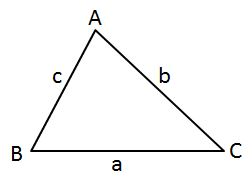#### Question 4 4. Find the value of x, rounded to the nearest tenth.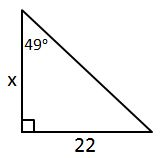### Page 2

#### Question 6 6. In the pictured triangle, ∠A is 98 degrees and ∠B is 12 degrees. If side a is 84 units long, approximately how long is side b?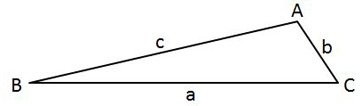#### Question 7 7. Find the value of x, rounded to the nearest degree.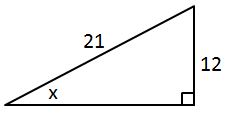#### Question 10 10. In the pictured triangle, side b is 12 and side c is 16. If ∠A is 84 degrees, then approximately how long is side a? Round the value of the cosine to the nearest whole number.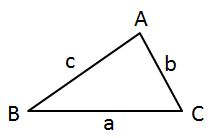### Page 3

#### Question 11 11. In the pictured triangle, ∠A is 58 degrees and ∠C is 63 degrees. If side a is 84 units long, approximately how long is side c?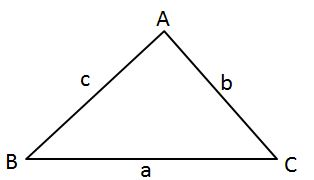### Page 4

#### Question 16 16. In the pictured triangle, side a is 23, side b is 18, and side c is 16. What is the approximate measure of ∠C? Round ∠C to the nearest whole number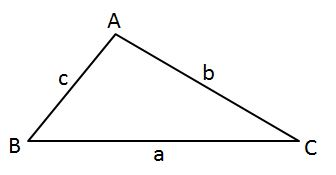#### Question 20 20. Simplify the following expression.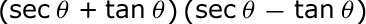### Page 5

#### Question 22 22. In the pictured triangle, ∠A is 62 degrees and ∠C is 86 degrees. If side a is 112 units long, approximately how long is side c?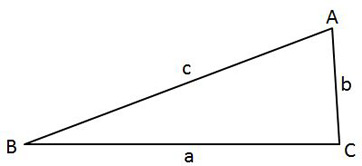#### Question 23 23. In the pictured triangle, side a is 9 and side b is 6. If ∠C is 26 degrees, then approximately how long is side c? Round the value of the cosine to the nearest whole number.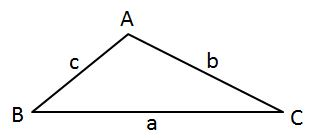#### Question 24 24. What's the value of 2sin(θ)cos(θ) given that the equation below is true?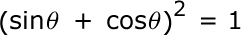#### Question 25 25. Find the value of x, rounded to the nearest tenth.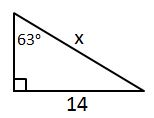### Page 6

#### Question 26 26. In the pictured triangle, ∠A is 137 degrees and ∠B is 28 degrees. If side b is 71 units long, approximately how long is side a?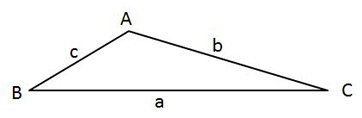#### Question 27 27. Find the value of x, rounded to the nearest tenth.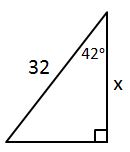#### Question 29 29. In the pictured triangle, what ratio represents the cosine of theta?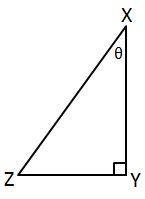#### Question 30 30. Find the value of x, rounded to the nearest degree.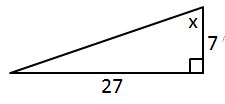#### Glencoe Geometry Chapter 7: Right Triangles and Trigonometry Chapter Exam Instructions

Choose your answers to the questions and click 'Next' to see the next set of questions. You can skip questions if you would like and come back to them later with the yellow "Go To First Skipped Question" button. When you have completed the practice exam, a green submit button will appear. Click it to see your results. Good luck!

Support